# Dividing money

Milan divided 360 euros in the ratio 4: 5, Erik in the ratio 500: 625. How many euros did the individual parts of Milan and how many Erik?

m1 =  160 Eur
m2 =  200 Eur
e1 =  160 Eur
e2 =  200 Eur

### Step-by-step explanation: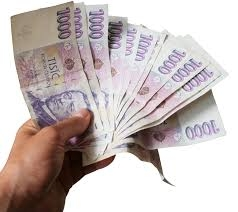Did you find an error or inaccuracy? Feel free to write us. Thank you!Tips to related online calculators
Need help to calculate sum, simplify or multiply fractions? Try our fraction calculator.
Check out our ratio calculator.

## Related math problems and questions:

• ErikThree friends Peter, Erik and Milan shared 420 euros in a ratio of 1: 2: 3. How many euros did Erik get?
• Ducats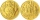The king divided the ducats to his three sons in a ratio of 2: 5: 4. How many ducats the king divided them if the youngest received 260 ducats, the least of all sons.
• TogetherThe three friends divided the balls in a ratio of 6: 5: 4. Some two of them got a total of 126 balls. How many balls were there together?
• Moneys in triple ratioMilan, John and Lili have a total 344 euros. Their amounts are in the ratio 1:2:5. Determine how much each of them has?
• Divide 5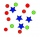Divide 288 in the following ratio 3 : 4 : 5
• Chess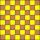How many ways can select 4 fields on a classic chessboard with 64 fields so that fields don't have the same color?
• The bookkeeperThe bookkeeper bought 45 copies of fairy tale books for CZK 120 and 65 copies of the encyclopedia for CZK 325. then he sold it in his shop like this: fairy tales for 135 CZK and encyclopedias for 360 CZK. What was the purchase price of the books? And the
• Word problem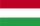348 students were on holiday in Hungary. 133 bought pizza, chips 28 pupils, 25 pupils bought soda, butter 26 pupils, 15 pupils fruits, vegetables 29 students. How much pay for all the food, if every meal cost 5.3 euros?
• Composite ratioJakub, Aneta, and Lenka divided 1342 USD in the ratio 5/2: 3/10: 1/4. How much did Lenka take?
• Four prismsQuestion No. 1: The prism has the dimensions a = 2.5 cm, b = 100 mm, c = 12 cm. What is its volume? a) 3000 cm2 b) 300 cm2 c) 3000 cm3 d) 300 cm3 Question No.2: The prism base is a rhombus with a side length of 30 cm and a height of 27 cm. The height of t
• Annual incomeThe annual income (in thousands of \$) of fifteen families is 60, 80, 90, 96, 120, 150, 200, 360, 480, 520, 1060, 1200, 1450, 2500, 7200. Calculate the harmonic and geometric mean.
• ŽSRCalculate fixed annual personnel costs of operating monorail line 118 km long if every 5 km is a station, which serves three people - one dispatcher and two switchmen in 4-shift operation. Consider the average salary of the employee 885 €.
• RemaindersIt is given a set of numbers { 170; 244; 299; 333; 351; 391; 423; 644 }. Divide this numbers by number 66 and determine set of remainders. As result write sum of this remainders.
• Three 43Three brothers inherited a cash amount of 62,000, and they divided it among themselves in the ratio of 5:4:1. How much more is the largest share than the smallest share?
• Divisible by 5How many three-digit odd numbers divisible by 5, which are in place ten's number 3?
• Birthday party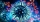For her youngest son's birthday party, the mother bought 6 3/4 kg of hotdog and 5 1/3 dozens bread rolls. Hotdogs cost 160 per kilogram, and a dozen bread rolls cost 25. How much did she spend in all?
• ConcertOn a Concert were sold 150 tickets for CZK 360, 235 tickets for 240 CZK and 412 for 180 CZK. How much was the total revenues for tickets?